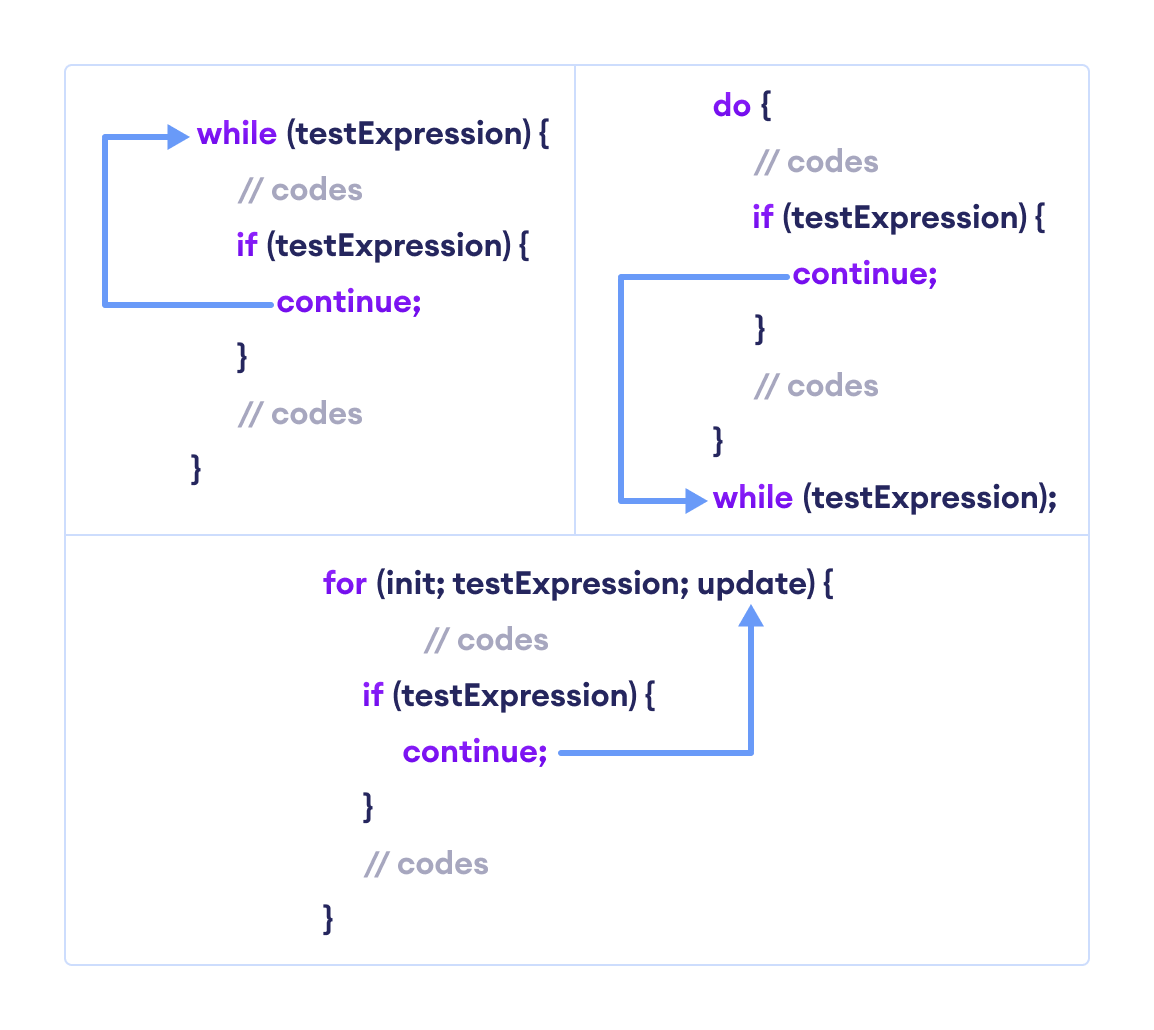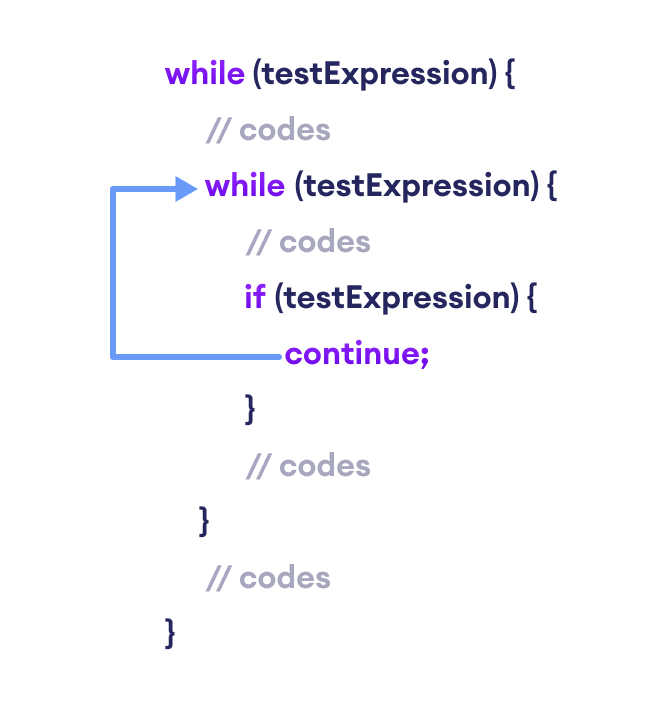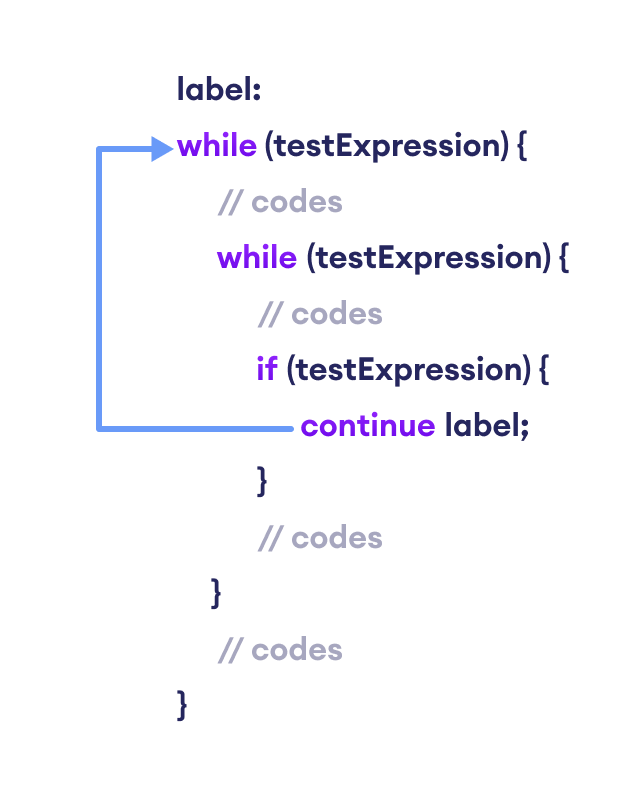# Java continue Statement

In this tutorial, you will learn about the continue statement and labeled continue statement in Java with the help of examples.

While working with loops, sometimes you might want to skip some statements or terminate the loop. In such cases, `break` and `continue` statements are used.

To learn about the `break` statement, visit Java break. Here, we will learn about the `continue` statement.

## Java continue

The `continue` statement skips the current iteration of a loop (`for`, `while`, `do...while`, etc).

After the `continue` statement, the program moves to the end of the loop. And, test expression is evaluated (update statement is evaluated in case of the for loop).

Here's the syntax of the continue statement.

``continue;``

Note: The continue statement is almost always used in decision-making statements (if...else Statement).

## Working of Java continue statementWorking of Java continue Statement

### Example 1: Java continue statement

``````class Main {
public static void main(String[] args) {

// for loop
for (int i = 1; i <= 10; ++i) {

// if value of i is between 4 and 9
// continue is executed
if (i > 4 && i < 9) {
continue;
}
System.out.println(i);
}
}
}``````

Output:

```1
2
3
4
9
10```

In the above program, we are using the `for` loop to print the value of i in each iteration. To know how `for` loop works, visit Java for loop. Notice the statement,

``````if (i > 5 && i < 9) {
continue;
}``````

Here, the `continue` statement is executed when the value of i becomes more than 4 and less than 9.

It then skips the print statement inside the loop. Hence we get the output with values 5, 6, 7, and 8 skipped.

### Example 2: Compute the sum of 5 positive numbers

``````import java.util.Scanner;

class Main {
public static void main(String[] args) {

Double number, sum = 0.0;
// create an object of Scanner
Scanner input = new Scanner(System.in);

for (int i = 1; i < 6; ++i) {
System.out.print("Enter number " + i + " : ");
// takes input from the user
number = input.nextDouble();

// if number is negative
// continue statement is executed
if (number <= 0.0) {
continue;
}

sum += number;
}
System.out.println("Sum = " + sum);
input.close();
}
}``````

Output:

```Enter number 1: 2.2
Enter number 2: 5.6
Enter number 3: 0
Enter number 4: -2.4
Enter number 5: -3
Sum = 7.8```

In the above example, we have used the for loop to print the sum of 5 positive numbers. Notice the line,

``````if (number < 0.0) {
continue;
}``````

Here, when the user enters a negative number, the `continue` statement is executed. This skips the current iteration of the loop and takes the program control to the update expression of the loop.

Note: To take input from the user, we have used the `Scanner` object. To learn more, visit Java Scanner.

## Java continue with Nested Loop

In the case of nested loops in Java, the `continue` statement skips the current iteration of the innermost loop.Working of Java continue statement with Nested Loops

### Example 3: continue with Nested Loop

``````class Main {
public static void main(String[] args) {

int i = 1, j = 1;

// outer loop
while (i <= 3) {

System.out.println("Outer Loop: " + i);

// inner loop
while(j <= 3) {

if(j == 2) {
j++;
continue;
}

System.out.println("Inner Loop: " + j);
j++;
}
i++;
}
}
}``````

Output

```Outer Loop: 1
Inner Loop: 1
Inner Loop: 3
Outer Loop: 2
Outer Loop: 3```

In the above example, we have used the nested while loop. Note that we have used the `continue` statement inside the inner loop.

``````if(j == 2) {
j++;
continue:
}``````

Here, when the value of j is 2, the value of j is increased and the `continue` statement is executed.

This skips the iteration of the inner loop. Hence, the text Inner Loop: 2 is skipped from the output.

## Labeled continue Statement

Till now, we have used the unlabeled `continue` statement. However, there is another form of `continue` statement in Java known as labeled continue.

It includes the label of the loop along with the `continue` keyword. For example,

``continue label;``

Here, the `continue` statement skips the current iteration of the loop specified by label.Working of the Java labeled continue Statement

We can see that the label identifier specifies the outer loop. Notice the use of the continue inside the inner loop.

Here, the `continue` statement is skipping the current iteration of the labeled statement (i.e. outer loop). Then, the program control goes to the next iteration of the labeled statement.

### Example 4: labeled continue Statement

``````class Main {
public static void main(String[] args) {

// outer loop is labeled as first
first:
for (int i = 1; i < 6; ++i) {

// inner loop
for (int j = 1; j < 5; ++j) {
if (i == 3 || j == 2)

// skips the current iteration of outer loop
continue first;
System.out.println("i = " + i + "; j = " + j);
}
}
}
}``````

Output:

```i = 1; j = 1
i = 2; j = 1
i = 4; j = 1
i = 5; j = 1```

In the above example, the `labeled continue` statement is used to skip the current iteration of the loop labeled as first.

``````if (i==3 || j==2)
continue first;``````

Here, we can see the outermost `for` loop is labeled as first,

``````first:
for (int i = 1; i < 6; ++i) {..}``````

Hence, the iteration of the outer `for` loop is skipped if the value of i is 3 or the value of j is 2.

Note: The use of labeled `continue` is often discouraged as it makes your code hard to understand. If you are in a situation where you have to use labeled `continue`, refactor your code and try to solve it in a different way to make it more readable.

Did you find this article helpful?